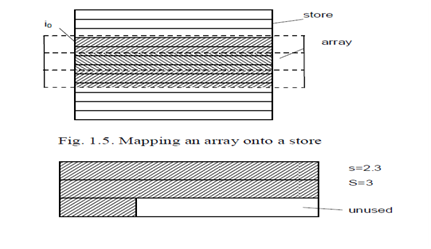## Representation of arrays?, Data Structure & Algorithms

Assignment Help:

A representation of an array structure is a mapping of the (abstract) array with elements of type T onto the store which is an array with elements of type BYTE. The array could be mapped in such a way that the computation of addresses of array elements is as simple as possible. The address i of the j-th array elements is computed by the linear mapping function

i = i0 + j*s

where i is the address of the first element, and s is the number of words that a element occupies. Assuming that the word is the smallest personally transferable unit of store, it is evidently highly needed that s be a whole number value, the simplest case being s = 1. If s is not a whole number, then s is generally rounded up to the next larger integer S. Each array component then accepts S words, whereby S-s words are left unused. Rounding up of the number of words required to the next whole number is called padding. The storage utilization factor u is the quotient of the minimal amounts of storage required to represent a structure and of the value actually used:#### Applications of linear and binary search, The searching method are applicab...

The searching method are applicable to a number of places in current's world, may it be Internet, search engines, text pattern matching, on line enquiry, finding a record from data

#### Storing a sparse matrix in memory, Explain an efficient method of storing a...

Explain an efficient method of storing a sparse matrix in memory. Write a module to find the transpose of the sparse matrix stored in this way. A matrix which contains number o

#### Notes, Ask question #Minimum 10000 words accepted#

Ask question #Minimum 10000 words accepted#

#### Convert graph into tree, How can we convert a graph into a tree ? Do we hav...

How can we convert a graph into a tree ? Do we have any standardized algorithm for doing this?

#### Non Recursive Algorithm to Traverse a Binary Tree, Q. Write down a non recu...

Q. Write down a non recursive algorithm to traverse a binary tree in order.                    Ans: N on - recursive algorithm to traverse a binary tree in inorder is as

#### Define the term - array, Define the term - Array A fixed length, ord...

Define the term - Array A fixed length, ordered collection of values of same type stored in contiguous memory locations; collection may be ordered in several dimensions.

#### Binry trees, Build a class ?Node?. It should have a ?value? that it stores ...

Build a class ?Node?. It should have a ?value? that it stores and also links to its parent and children (if they exist). Build getters and setters for it (e.g. parent node, child n

#### Entity relationship diagram, This question is based on the requirements of ...

This question is based on the requirements of a system to record band bookings at gigs. (A 'gig' is an event at which one or more bands are booked to play). You do not need to know

#### Determine the components of illumination, Determine the Components of Illum...

Determine the Components of Illumination The light reaching the eye when looking at a surface has clearly come from a source (or sources) of illumination and bounced off the su

#### Adjacency matrix of an undirected graph, 1) What will call a graph that hav...

1) What will call a graph that have no cycle? 2) Adjacency matrix of an undirected graph is------------- on main diagonal. 3) Represent the following graphs by adjacency matr